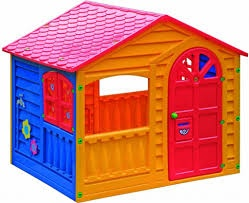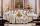# Base of house

Calculate the volume of the bases of a square house, if the base depth is 1.2 m, the width is 40 cm and their outer circumference is 40.7 m.

Correct result:

V =  18.768 m3

#### Solution:

$h=1.2 \ \text{m} \ \\ s=40 \ cm \rightarrow m=40 / 100 \ m=0.4 \ m \ \\ o=40.7 \ \text{m} \ \\ \ \\ a=o/4=40.7/4=\dfrac{ 407 }{ 40 }=10.175 \ \text{m} \ \\ b=a - 2 \cdot \ s=10.175 - 2 \cdot \ 0.4=\dfrac{ 75 }{ 8 }=9.375 \ \text{m} \ \\ \ \\ S_{1}=a^2=10.175^2 \doteq 103.5306 \ \text{m}^2 \ \\ S_{2}=b^2=9.375^2 \doteq \dfrac{ 5625 }{ 64 } \doteq 87.8906 \ \text{m}^2 \ \\ \ \\ S=S_{1}-S_{2}=103.5306-87.8906=\dfrac{ 391 }{ 25 }=15.64 \ \text{m}^2 \ \\ V=S \cdot \ h=15.64 \cdot \ 1.2=\dfrac{ 2346 }{ 125 }=18.768 \ \text{m}^3$Our examples were largely sent or created by pupils and students themselves. Therefore, we would be pleased if you could send us any errors you found, spelling mistakes, or rephasing the example. Thank you!

Please write to us with your comment on the math problem or ask something. Thank you for helping each other - students, teachers, parents, and problem authors.Tips to related online calculators
Do you want to convert length units?
Do you know the volume and unit volume, and want to convert volume units?

#### You need to know the following knowledge to solve this word math problem:

We encourage you to watch this tutorial video on this math problem:

## Next similar math problems:

• Four prismsQuestion No. 1: The prism has the dimensions a = 2.5 cm, b = 100 mm, c = 12 cm. What is its volume? a) 3000 cm2 b) 300 cm2 c) 3000 cm3 d) 300 cm3 Question No.2: The base of the prism is a rhombus with a side length of 30 cm and a height of 27 cm. The heigThe surface of the regular quadrilateral prism is 8800 cm2, the base edge is 20 cm long. Calculate the volume of the prismCalculate the volume (V) and the surface (S) of a regular quadrilateral prism whose height is 28.6 cm and the deviation of the body diagonal from the base plane is 50°.
• Prism 4 sidesThe prism has a square base with a side length of 3 cm. The diagonal of the sidewall of the prism/BG/is 5 cm. Calculate the surface of this prism in cm square and the volume in liters
• Angle of diagonalAngle between the body diagonal of a regular quadrilateral and its base is 60°. The edge of the base has a length of 10cm. Calculate the body volume.Calculate the volume and surface area of a regular quadrangular prism 35 cm high and the base diagonal of 22 cm.
• Alien shipThe alien ship has the shape of a sphere with a radius of r = 3000m, and its crew needs the ship to carry the collected research material in a cuboid box with a square base. Determine the length of the base and (and height h) so that the box has the large
• Prism diagonalThe body diagonal of a regular square prism has an angle of 60 degrees with the base, the edge length is 10 cm. What is the volume of the prism?
• Regular prismThe regular four-sided prism has a base of 25 cm2 and a surface of 210 cm2. Find its volume.
• Find theFind the volume of a quadrangle prism high 2dm whose base is a square with a side 15cm.
• Cutting the prismA prism with a square base with a content of 1 cm2 and a height of 3 cm was cut from a cube with an edge length of 3 cm. What is the surface of the body formed from the cube after cutting the prism?
• Square prismA square prism has a base with a length of 23 centimeters, what is the area in square centimeters of the base of the prism?
• Edge of prismThe regular quadrilateral prism has a surface of 250 dm2, its shell has a content of 200 dm2. Calculate its leading edge.
• Cube cutIn the ABCDA'B'C'D'cube, it is guided by the edge of the CC' a plane witch dividing the cube into two perpendicular four-sided and triangular prisms, whose volumes are 3:2. Determine in which ratio the edge AB is divided by this plane.
• The aquariumThe aquarium has a capacity of 18 liters. What is its height when the square bottom is 8 2/3 cm long?
• Density of the concreteFind the density of the concrete of the cuboid-shaped column has dimensions of 20 x 20 cm x 2 m if the weight of the column is 200 kg.
• Iron poleWhat is the mass of pole with the shape of a regular quadrilateral prism with a length of 1 m and a cross-sectional side length of a = 4.5 cm make from iron with density ρ = 7800 kg/m³?